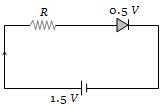NEET Physics Semiconductor Electronics Questions Solved

The diode used in the circuit shown in the figure has a constant voltage drop of 0.5 V at all currents and a maximum power rating of 100 milliwatts. What should be the value of the resistor R, connected in series with the diode for obtaining maximum current(a) 1.5 $\mathrm{\Omega }$
(b) 5 $\mathrm{\Omega }$
(c) 6.67 $\mathrm{\Omega }$
(d) 200 $\mathrm{\Omega }$

Explanation is a part of a Paid Course. To view Explanation Please buy the course.

Difficulty Level: